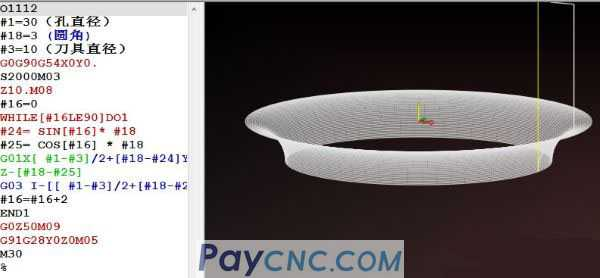Today, take the machining center round arc R as an example (using a standard milling cutter), as shown in the figure below:Faced with this part, many young masters do not know how to start. Zou Jun, I once again emphasize that the case is not important, because there are cases everywhere, and it is more important to pass on the ideas and methods of writing to you.   So in this article, you can learn the following points:   1. How to calculate point coordinates.   2. Derivation of mathematical relations.   3. Use mathematical relations to complete the editing of the macro program.     1. How to calculate point coordinates.   We know that whether it is software programming or manual programming, a program is nothing more than two major components:   1. G command. 2. Point coordinates.   There are only dozens of CNC G commands commonly used, a few, but whether the part is a straight line, an arc, or a curved surface, it is composed of countless small points, and then the points are connected by small line segments. , Which constitutes a wide range of products.   So how to deal with countless point data, once again, software programming is a tool, and macro programming is also a tool. Since it is a tool, you can use that which is simple.   For example, if the orifice is reversed to R, you can handle it with software. It is very simple to understand macro programming, and it can be done in a few sentences, and the versatility is also very good. Many small partners, using framework programming, can quickly complete the programming of the program,   Ok, I will focus on explaining how to calculate the point coordinates on the arc.   For example, the following is an enlarged schematic diagram of arc R.I set an arbitrary point P on the arc and drew a right triangle, so that using the Pythagorean theorem, I can find the #24 and #25 of the point P   The formula of the Pythagorean theorem is as follows: SIN[#16]=#24/#18 COS[#16]=#25/#18   However, if #24 and #25 are required, you need to know the data of the other two variables, and #18 is the arc radius, which belongs to the known data.   #16 is the set angle variable, then the variable #16 can be incremented (0 degrees -90 degrees), so that the data of #24 and #25 are calculated.     2. Derivation of mathematical relations.   You will find that #24 and #25 are data centered on the arc R. The point coordinates in the program are calculated based on the programmed origin. For example, in the figure below, the hole center is the programming origin.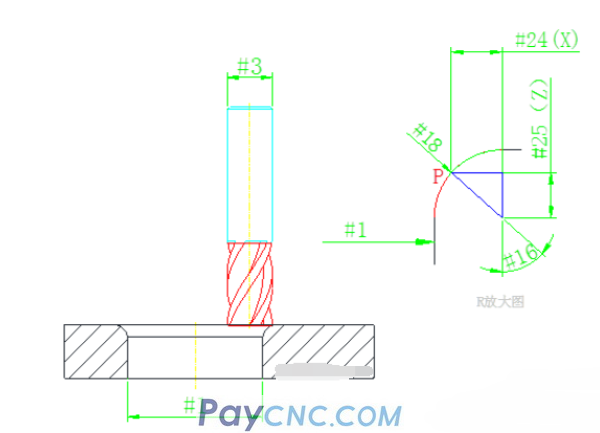Further analysis, you will easily calculate the following formula:   X direction: [#1-#3]/2+[#18-#24]   Z direction: #18-#25   A picture is worth a thousand words. If you look at the above picture and the variables carefully, the relationship between the variables can be easily derived.     3. Use mathematical relations to complete the editing of the macro program.   The key to learning thoroughly is to learn by analogy. Friends who have seen my macro programming explanation may know that it is not difficult to write programs using mathematical formulas.   For example, hyperbola, quadratic equation, parabola, curve, etc., because there are ready-made formulas, aren't the X, Y, and Z in the "formula" exactly the point coordinates we require?   For example, the curve in the figure below needs to be processed on a CNC car, and the curve equation is: Z=32-X²/8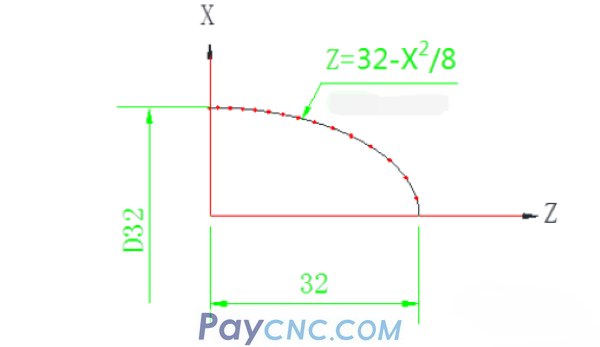Then give X a value, then there will be a corresponding Z value, X and Z satisfy the equation. Set the independent variable and dependent variable, and at the same time complete the editing of the program quickly through the self-increment of the variable.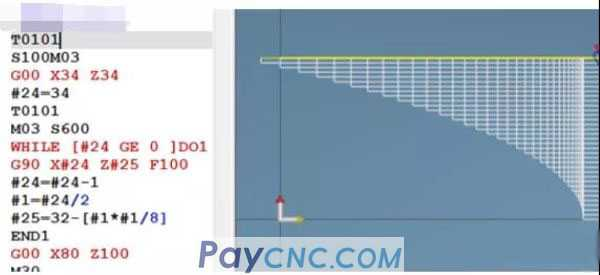Ellipse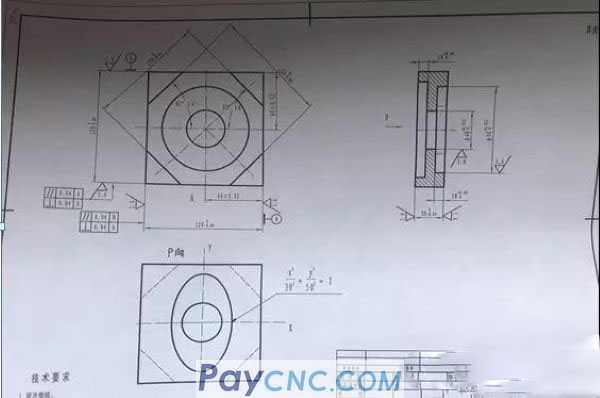The ellipse equation is: X²/a²+Y²/b²=1 Give X a value, then there will be a corresponding Y value, X and Y satisfy the elliptic equation. Set the independent variable and dependent variable, and at the same time complete the editing of the program quickly through the self-increment of the variable.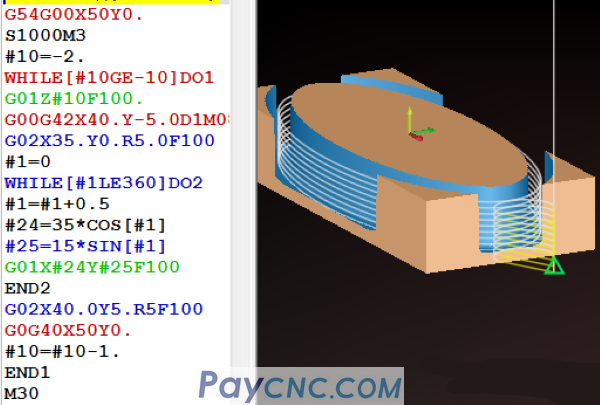For example, round the arc of the orifice mentioned at the beginning of the article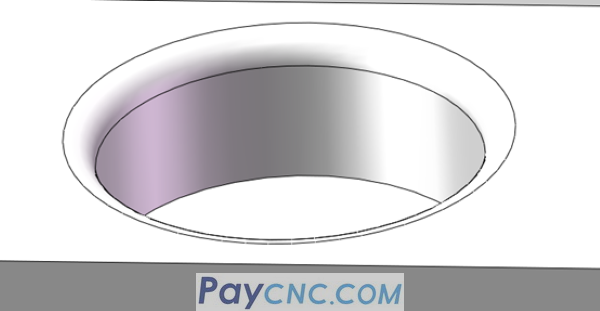Using the Pythagorean law and the derived relational expressions, the editing of the program is completed in a few sentences.